# 71 Results

View
Selected filters:
• Mathematics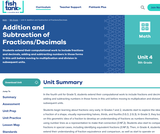Conditional Remix & Share Permitted
CC BY-NC-SA
Rating

Students extend their computational work to include fractions and decimals, adding and subtracting numbers in those forms in this unit before moving to multiplication and division in subsequent units.

Subject:
Mathematics
Material Type:
Unit of Study
Provider:
Fishtank Learning
Provider Set:
Mathematics
11/19/2021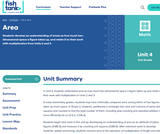Conditional Remix & Share Permitted
CC BY-NC-SA
Rating

Students develop an understanding of areas as how much two-dimensional space a figure takes up, and relate it to their work with multiplication from Units 2 and 3.

Subject:
Mathematics
Material Type:
Unit of Study
Provider:
Fishtank Learning
Provider Set:
Mathematics
11/19/2021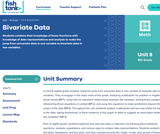Conditional Remix & Share Permitted
CC BY-NC-SA
Rating

Students combine their knowledge of linear functions with knowledge of data representations and analysis to make the jump from univariate data in one variable to bivariate data in two variables.

Subject:
Mathematics
Material Type:
Unit of Study
Provider:
Fishtank Learning
Provider Set:
Mathematics
11/19/2021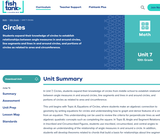Conditional Remix & Share Permitted
CC BY-NC-SA
Rating

Students expand their knowledge of circles to establish relationships between angle measures in and around circles, line segments and lines in and around circles, and portions of circles as related to area and circumference.

Subject:
Mathematics
Geometry
Material Type:
Unit of Study
Provider:
Fishtank Learning
Provider Set:
Mathematics
11/19/2021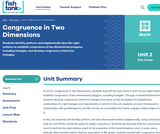Conditional Remix & Share Permitted
CC BY-NC-SA
Rating

Students identify, perform, and algebraically describe rigid motions to establish congruence of two dimensional polygons, including triangles, and develop congruence criteria for triangles.

Subject:
Mathematics
Geometry
Material Type:
Unit of Study
Provider:
Fishtank Learning
Provider Set:
Mathematics
11/19/2021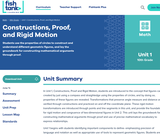Conditional Remix & Share Permitted
CC BY-NC-SA
Rating

Students use the properties of circles to construct and understand different geometric figures, and lay the groundwork for constructing mathematical arguments through proof.

Subject:
Mathematics
Geometry
Material Type:
Unit of Study
Provider:
Fishtank Learning
Provider Set:
Mathematics
11/19/2021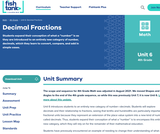Conditional Remix & Share Permitted
CC BY-NC-SA
Rating

Students expand their conception of what a “number” is as they are introduced to an entirely new category of number, decimals, which they learn to convert, compare, and add in simple cases.

Subject:
Mathematics
Material Type:
Unit of Study
Provider:
Fishtank Learning
Provider Set:
Mathematics
11/19/2021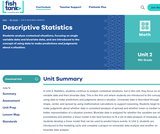Conditional Remix & Share Permitted
CC BY-NC-SA
Rating

Students analyze contextual situations, focusing on single variable data and bivariate data, and are introduced to the concept of using data to make predictions and judgments about a situation.

Subject:
Mathematics
Algebra
Material Type:
Unit of Study
Provider:
Fishtank Learning
Provider Set:
Mathematics
11/19/2021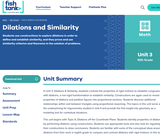Conditional Remix & Share Permitted
CC BY-NC-SA
Rating

Students use constructions to explore dilations in order to define and establish similarity, and they prove and use similarity criterion and theorems in the solution of problems.

Subject:
Mathematics
Geometry
Material Type:
Unit of Study
Provider:
Fishtank Learning
Provider Set:
Mathematics
11/19/2021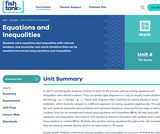Conditional Remix & Share Permitted
CC BY-NC-SA
Rating

Students solve equations and inequalities with rational numbers, and encounter real-world situations that can be modeled and solved using equations and inequalities.

Subject:
Mathematics
Material Type:
Unit of Study
Provider:
Fishtank Learning
Provider Set:
Mathematics
11/19/2021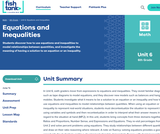Conditional Remix & Share Permitted
CC BY-NC-SA
Rating

Students discover how to use equations and inequalities to model relationships between quantities, and investigate the meaning of having a solution to an equation or an inequality.

Subject:
Mathematics
Material Type:
Unit of Study
Provider:
Fishtank Learning
Provider Set:
Mathematics
11/19/2021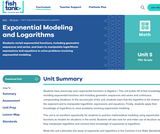Conditional Remix & Share Permitted
CC BY-NC-SA
Rating

Students revisit exponential functions, including geometric sequences and series, and learn to manipulate logarithmic expressions and equations to solve problems involving exponential modeling.

Subject:
Mathematics
Algebra
Material Type:
Unit of Study
Provider:
Fishtank Learning
Provider Set:
Mathematics
11/19/2021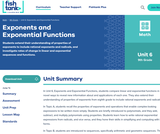Conditional Remix & Share Permitted
CC BY-NC-SA
Rating

Students extend their understanding of properties of exponents to include rational exponents and radicals, and investigate rates of change in linear and exponential sequences and functions.

Subject:
Mathematics
Algebra
Material Type:
Unit of Study
Provider:
Fishtank Learning
Provider Set:
Mathematics
11/19/2021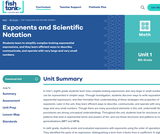Conditional Remix & Share Permitted
CC BY-NC-SA
Rating

Students learn to simplify complex-looking exponential expressions, and they learn efficient ways to describe, communicate, and operate with very large and very small numbers.

Subject:
Mathematics
Material Type:
Unit of Study
Provider:
Fishtank Learning
Provider Set:
Mathematics
11/19/2021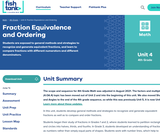Conditional Remix & Share Permitted
CC BY-NC-SA
Rating

Students are exposed to general methods and strategies to recognize and generate equivalent fractions, and learn to compare fractions with different numerators and different denominators.

Subject:
Mathematics
Material Type:
Unit of Study
Provider:
Fishtank Learning
Provider Set:
Mathematics
11/19/2021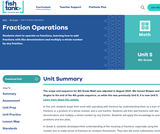Conditional Remix & Share Permitted
CC BY-NC-SA
Rating

Students start to operate on fractions, learning how to add fractions with like denominators and multiply a whole number by any fraction.

Subject:
Mathematics
Material Type:
Unit of Study
Provider:
Fishtank Learning
Provider Set:
Mathematics
11/19/2021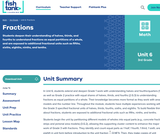Conditional Remix & Share Permitted
CC BY-NC-SA
Rating

Students deepen their understanding of halves, thirds, and fourths to understand fractions as equal partitions of a whole, and are exposed to additional fractional units such as fifths, sixths, eighths, ninths, and tenths.

Subject:
Mathematics
Material Type:
Unit of Study
Provider:
Fishtank Learning
Provider Set:
Mathematics
11/19/2021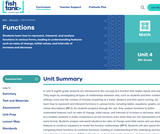Conditional Remix & Share Permitted
CC BY-NC-SA
Rating

Students learn how to represent, interpret, and analyze functions in various forms, leading to understanding features such as rates of change, initial values, and intervals of increase and decrease.

Subject:
Mathematics
Material Type:
Unit of Study
Provider:
Fishtank Learning
Provider Set:
Mathematics
11/19/2021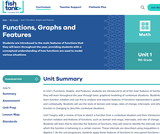Conditional Remix & Share Permitted
CC BY-NC-SA
Rating

Students are introduced to the main features of functions that they will learn throughout the year, providing students with a conceptual understanding of how functions are used to model various situations.

Subject:
Mathematics
Algebra
Material Type:
Unit of Study
Provider:
Fishtank Learning
Provider Set:
Mathematics
11/19/2021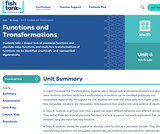Conditional Remix & Share Permitted
CC BY-NC-SA
Rating

Students take a deeper look at piecewise functions and absolute value functions, and study how transformations of functions can be identified graphically and represented algebraically.

Subject:
Mathematics
Algebra
Material Type:
Unit of Study
Provider:
Fishtank Learning
Provider Set:
Mathematics